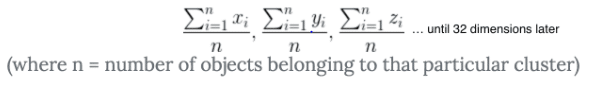1. 一些代码, 显示如何使用 K 手段。
2. 为什么不应该使用 K 手段进行上下文时间序列异常检测。

一些代码显示它是如何使用的

K 意味着会形成集群。但如何？时间序列数据看起来不像一个美丽的散布情节, 是 “群集”。对数据进行窗口化会获取类似于此的数据.。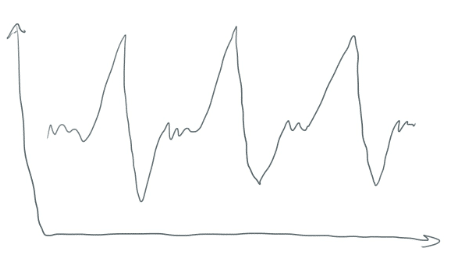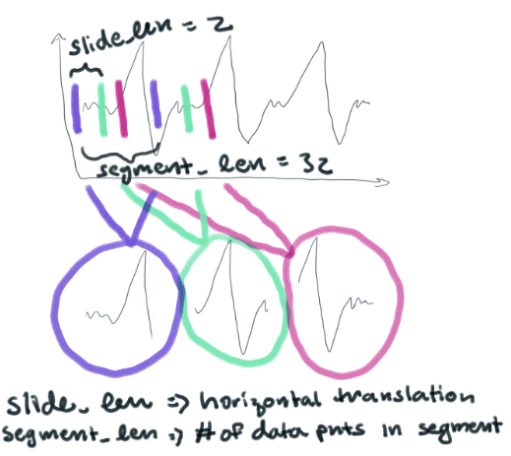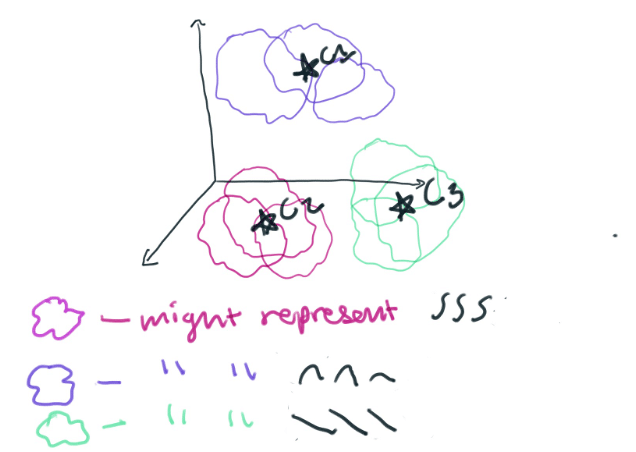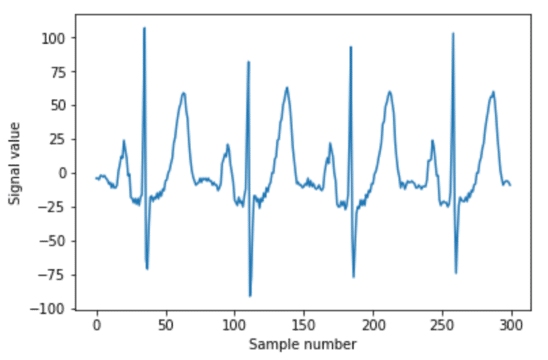``````import numpy as np

segment_len = 32
slide_len = 2

segments = []
for start_pos in range(0, len(ekg_data), slide_len):
end_pos = start_pos + segment_len
segment = np.copy(ekg_data[start_pos:end_pos])
# if we're at the end and we've got a truncated segment, drop it
if len(segment) != segment_len:
continue
segments.append(segment)``````window_rads = np.linspace(0, np.pi, segment_len) window = np.sin(window_rads)**2 windowed_segments = [] for segment in segments: windowed_segment = np.copy(segment) * window windowed_segments.append(windowed_segment)

2. 分组时间

``````from sklearn.cluster import KMeans

clusterer = KMeans(n_clusters=150)
clusterer.fit(windowed_segments)``````

3. 重建时间

``````#placeholder reconstruction array
reconstruction = np.zeros(len(ekg_data_anomalous))``````

``````slide_len = segment_len/2
# slide_len = 16 as opposed to a slide_len = 2. Slide_len = 2 was used to create a lot of horizontal translations to provide K-Means with a lot of data.

#segments were created from the ekg_data_anomalous dataset from the code above
for segment_n, segment in enumerate(segments):
# normalize by multiplying our window function to each segment
segment *= window
# sklearn uses the euclidean square distance to predict the centroid
nearest_centroid_idx = clusterer.predict(segment.reshape(1,-1))
centroids = clusterer.cluster_centers_
nearest_centroid = np.copy(centroids[nearest_centroid_idx])

# reconstructed our segments with an overlap equal to the slide_len so the centroids are
stitched together perfectly.
pos = segment_n * slide_len
reconstruction[int(pos):int(pos+segment_len)] += nearest_centroid``````

``````error = reconstruction[0:n_plot_samples] - ekg_data_anomalous[0:n_plot_samples]
error_98th_percentile = np.percentile(error, 98)``````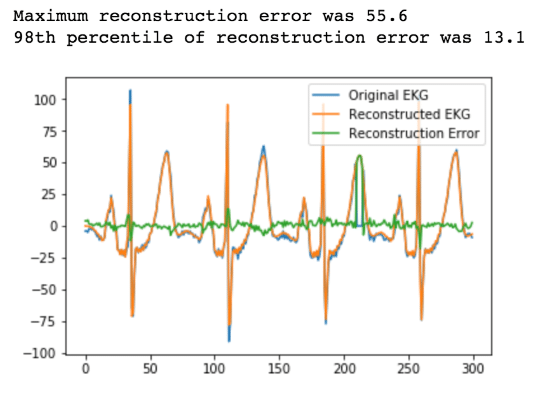4. 警报为什么不应该使用 K 手段进行上下文时间序列异常检测